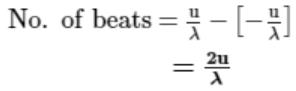Courses

# Test: Beats

## 10 Questions MCQ Test Physics Class 11 | Test: Beats

Description
This mock test of Test: Beats for JEE helps you for every JEE entrance exam. This contains 10 Multiple Choice Questions for JEE Test: Beats (mcq) to study with solutions a complete question bank. The solved questions answers in this Test: Beats quiz give you a good mix of easy questions and tough questions. JEE students definitely take this Test: Beats exercise for a better result in the exam. You can find other Test: Beats extra questions, long questions & short questions for JEE on EduRev as well by searching above.
QUESTION: 1

### Two guitar strings A and B are slightly out of tune and produce beats of frequency 6Hz.The tension of the string found to decrease to 4 Hz. What is the original frequency of B if the frequency of a is 430Hz?

Solution:

Answer :- a

Solution :- Let original freq. of sitar string A be na and original freq. of sitar string B be nb

As number of beats/ sec. = 6

∴ nb = na ± 6=430±6=436 or 424Hz

When tension in A is reduced, its frequency reduces

(∵n∝√T)

As number of beats /sec decreases to 4 therefore, frequency of

B=430−6=424Hz

QUESTION: 2

### When two tuning forks are sounded together 4 beats are heard per second. One tuning fork is of frequency 346 Hz. When its prong is loaded with a little wax, the number of beats is increased to 6 per second. The frequency of the other fork is:

Solution:

Frequency of fork one is 346 Hz.
When the other fork is waxed, the beat is increased.
So, the frequency of other fork is less than the frequency of fork one.
So,
beat = frequency of fork one − frequency of second fork
4=346− Frequency of second fork
Frequency of second fork =350 Hz

QUESTION: 3

### What material is tuning fork made of and why?

Solution:

A tuning fork is made of an alloy of steel, nickel and chromium, called elinvar, i.e. the material for which the elasticity does not change.

QUESTION: 4

Phenomenon of beats is not used in

Solution:

Radar uses electromagnetic energy pulses. The radio-frequency (rf) energy is transmitted to and reflected from the reflecting object. A small portion of the reflected energy returns to the radar set. This returned energy is called an ECHO. Radar sets use the echo to determine the direction and distance of the reflecting object.
It does not work on phenomena of beats.

QUESTION: 5

For the formation of distinct beats, the frequencies of two sources of sound should be

Solution:

For the formation of distinct beats, frequencies of two sources of sound should be nearly equal, i.e., difference in frequencies of two sources must be small, say less than 10. The impression of sound heard by ow ears persists on our mind for 1 /10th of a second. If another sound is heard before (1 /10) second passes, the impressions of the two sounds mix up and our mind cannot distinguish between the two. In order to hear distinct beats, time interval between two successive beats must be greater than 1 /10 second. Therefore, frequency of beats must be less than 1 0,i.e., number of beats/sec, which is equal to difference in frequencies of two sources must be less than 10. Hence the two sources should be of nearly equal frequencies.

QUESTION: 6

On adding two sine waves that are exactly the same, what would be the result?

Solution:

According to the Principle of Superposition, if the particle of a medium is given an order (i.e. of oscillating sinusoidally) and then another order (similar or different), the particle simply adds the two orders vectorially (i.e. by vector method), and then oscillates by the amplitude of the resultant vector.
So if the particle of a medium is given two similar orders (i.e., both the sine waves having the same amplitude, say A) then the particle will oscillate with an amplitude, 2A, i.e., the amplitude will be doubled.

QUESTION: 7

Time interval between two successive maxima of sound waves with frequencies v1 and v2 is given by

Solution:

As we know time interval will have dimensions of time so check in option for dimension of time.
Dimensions of v is "per second" or [T-1]

QUESTION: 8

Two sound sources fixed at a given distance apart are emitting sound each of wavelength λ. A Listener moves with a velocity v0 along the line joining the two sources , the number of beats heard by him per second is

Solution:

Number extra waves received from each side:

n = +- u/λ

where

u= speed of the observer

λ = Wavelength of sound

Number of beats:The number of beats is given by the difference between number of extra waves received on either sides.QUESTION: 9

Pure sound notes from two sources make the molecules of air at a location vibrate simple harmonically in accordance with the equations.
y1 = 0.008 sin (604 n t) and
y2 = 0.007 sin (610 n t) respectively.
The number of beast heard by a person at the location will be:

Solution:
QUESTION: 10

Two tuning forks are struck and the sounds from each reach your ears at the same time. One sound has a frequency of 256 Hz, and the second sound has a frequency of 258 Hz. The underlying beat frequency is:

Solution:

The beat frequency is always equal to the difference in frequency of the two notes that interfere to produce the beats. So if two sound waves with frequencies of 258 Hz and 256 Hz are played simultaneously, a beat frequency of 2 Hz will be detected.

• Test